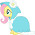# Online Wh to mAh Converter

Just a useful little utility :)

 Wh (Eg: 4.44): V (Eg: 3.7): mAh:

Formula: mAh = Wh * 1000 / V

<script type="text/javascript">
function convertWhtomAh()
{
var WhValue = document.getElementById('convertWh').value;
var VValue= document.getElementById('convertV').value;
var result = (WhValue * 1000) / VValue;
void(document.getElementById('convertmAh').value = result);
}
</script>

#### 3 comments :

1.You may want to check the math

1.Seems correct - What problems are you having?

The example numbers give 1200, which is correct.

2.Thanks, exactly what I needed